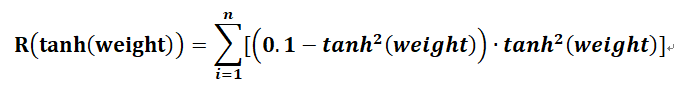# How to implement a customize weight

Hi, I would like to implement tanh(weight) instead of weight, for example:
I am not sure if there is a problem with my code，so thank you so much for your help.

``````class LeNet(nn.Module):
def __init__(self):
super(LeNet, self).__init__()
self.conv1 = nn.Sequential(
nn.Conv2d(1, 6, 3, 1, 2,bias=False),
nn.ReLU(),
nn.MaxPool2d(2, 2)
)
self.conv2 = nn.Sequential(
nn.Conv2d(6, 16, 5,bias=False),
nn.ReLU(),
nn.MaxPool2d(2, 2)
)
self.fc1 = nn.Linear(16 * 5 * 5, 120,bias=False)
self.fc2 = nn.Linear(120, 10,bias=False)
self.conv1.weight =torch.tanh(self.conv1.weight)
self.conv2.weight =torch.tanh(self.conv2.weight)
self.fc1.weight =torch.tanh(self.fc1.weight)
self.fc2.weight =torch.tanh(self.fc2.weight)

def forward(self, x):
x = self.conv1(x)
x = self.conv2(x)
x = x.view(x.size(), -1)
x = self.fc1(x)
x= F.relu(x)
x = self.fc2(x)
return x
``````

Do you mean `weight` is learnable and calculate `y = tanh(weight) * x` when learning?

``````class LeNet(nn.Module):
def __init__(self):
super(LeNet, self).__init__()
self.conv1 = nn.Sequential(
nn.Conv2d(1, 6, 3, 1, 2,bias=False),
nn.ReLU(),
nn.MaxPool2d(2, 2)
)
self.conv2 = nn.Sequential(
nn.Conv2d(6, 16, 5,bias=False),
nn.ReLU(),
nn.MaxPool2d(2, 2)
)
self.fc1 = nn.Linear(16 * 5 * 5, 120,bias=False)
self.fc2 = nn.Linear(120, 10,bias=False)

def forward(self, x):
self.conv1.weight[:] = torch.tanh(self.conv1.weight[:])
self.conv2.weight[:] = torch.tanh(self.conv2.weight[:])
self.fc1.weight[:] = torch.tanh(self.fc1.weight[:])
self.fc2.weight[:] = torch.tanh(self.fc2.weight[:])
x = self.conv1(x)
x = self.conv2(x)
x = x.view(x.size(), -1)
x = self.fc1(x)
x= F.relu(x)
x = self.fc2(x)
return x
``````

I am not sure `with torch.no_grad` is needed or not.

1 Like

Thank you for your kind reply. Yes, weight is a learnable parameter, and use tanh(weight) to limit the weight range.
Apart from `with torch.no_grad`, what is the difference between putting `self.conv1.weight[:] = torch.tanh(self.conv1.weight[:])` before `def forward(self, x):` and inside `def forward(self, x):`.

Thank you again for your time.
Best regards.

`__init__` only work when you init your network.
If you want to limit it, inside `forward`
.

oh! Thank you so much!

If all you want is to limit the values to be in a range, why not using the torch.clamp?
I just want to make sure you use the right tools here, `tanh` is an activation function, and it transforms your input to be in that range, depending on the task it might not give you what you desire.
So if you know what you are doing then fine, in case you didn’t know, check `torch.clamp` out, maybe this is what you were looking for?

Yes, if ONLY CLIP, check here

1 Like

Hi, thank you for your suggestion. I have tried` torch.clamp`, besides, I also want to use `tanh (weight)` for `weight regularizer` for loss function, for example:Thank you so much for all.

1 Like